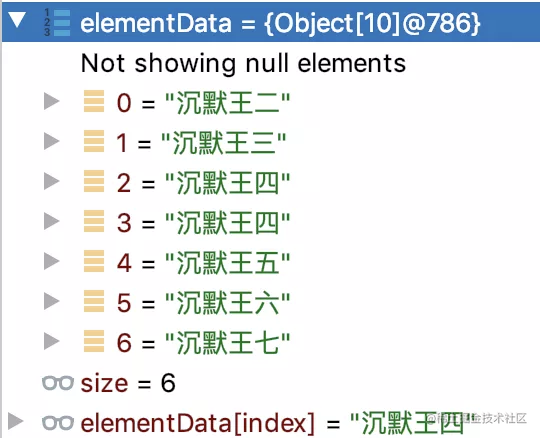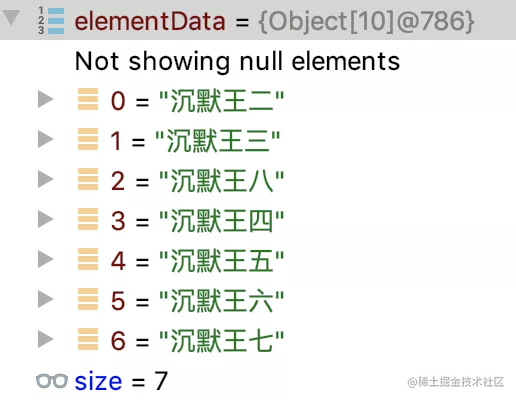github.com/itwanger/to…

1）get(int index) 方法的时间复杂度为 $O(1)$，因为是直接从底层数组根据下标获取的，和数组长度无关。

public E get(int index) {
Objects.checkIndex(index, size);
return elementData(index);
}

2）add(E e) 方法会默认将元素添加到数组末尾，但需要考虑到数组扩容的情况，如果不需要扩容，时间复杂度为 $O(1)$

public boolean add(E e) {
modCount++;
return true;
}

private void add(E e, Object[] elementData, int s) {
if (s == elementData.length)
elementData = grow();
elementData[s] = e;
size = s + 1;
}

private Object[] grow(int minCapacity) {
int oldCapacity = elementData.length;
if (oldCapacity > 0 || elementData != DEFAULTCAPACITY_EMPTY_ELEMENTDATA) {
int newCapacity = ArraysSupport.newLength(oldCapacity,
minCapacity - oldCapacity, /* minimum growth */
oldCapacity >> 1           /* preferred growth */);
return elementData = Arrays.copyOf(elementData, newCapacity);
} else {
return elementData = new Object[Math.max(DEFAULT_CAPACITY, minCapacity)];
}
}

3）add(int index, E element) 方法将新的元素插入到指定的位置，考虑到需要复制底层数组（根据之前的判断，扩容的话，数组可能要复制一次），根据最坏的打算（不管需要不需要扩容，System.arraycopy() 肯定要执行），所以时间复杂度为 $O(n)$

public void add(int index, E element) {
modCount++;
final int s;
Object[] elementData;
if ((s = size) == (elementData = this.elementData).length)
elementData = grow();
System.arraycopy(elementData, index,
elementData, index + 1,
s - index);
elementData[index] = element;
size = s + 1;
}

ArrayList<String> list = new ArrayList<>();

System.arraycopy() 执行完成后，下标为 2 的元素为沉默王四，这一点需要注意。也就是说，在数组中插入元素的时候，会把插入位置以后的元素依次往后复制，所以下标为 2 和下标为 3 的元素都为沉默王四。4） remove(int index) 方法将指定位置上的元素删除，考虑到需要复制底层数组，所以时间复杂度为 $O(n)$

public E remove(int index) {
Objects.checkIndex(index, size);
final Object[] es = elementData;

@SuppressWarnings("unchecked") E oldValue = (E) es[index];
fastRemove(es, index);

return oldValue;
}
private void fastRemove(Object[] es, int i) {
modCount++;
final int newSize;
if ((newSize = size - 1) > i)
System.arraycopy(es, i + 1, es, i, newSize - i);
es[size = newSize] = null;
}

1）get(int index) 方法的时间复杂度为 $O(n)$，因为需要循环遍历整个链表。

public E get(int index) {
checkElementIndex(index);
return node(index).item;
}

// assert isElementIndex(index);

if (index < (size >> 1)) {
for (int i = 0; i < index; i++)
x = x.next;
return x;
} else {
for (int i = size - 1; i > index; i--)
x = x.prev;
return x;
}
}

public E getFirst() {
if (f == null)
throw new NoSuchElementException();
return f.item;
}

public E getLast() {
if (l == null)
throw new NoSuchElementException();
return l.item;
}

first 和 last 在链表中是直接存储的，所以时间复杂度为 $O(1)$

2）add(E e) 方法默认将元素添加到链表末尾，所以时间复杂度为 $O(1)$

public boolean add(E e) {
return true;
}
last = newNode;
if (l == null)
first = newNode;
else
l.next = newNode;
size++;
modCount++;
}

3）add(int index, E element) 方法将新的元素插入到指定的位置，需要先通过遍历查找这个元素，然后再进行插入，所以时间复杂度为 $O(n)$

public void add(int index, E element) {
checkPositionIndex(index);

if (index == size)
else
}

public void addFirst(E e) {
}
first = newNode;
if (f == null)
last = newNode;
else
f.prev = newNode;
size++;
modCount++;
}

linkFirst() 只需要对 first 进行更新即可。

public void addLast(E e) {
}

last = newNode;
if (l == null)
first = newNode;
else
l.next = newNode;
size++;
modCount++;
}

linkLast() 只需要对 last 进行更新即可。

void linkBefore(E e, LinkedList.Node<E> succ) {
// assert succ != null;
succ.prev = newNode;
if (pred == null)
first = newNode;
else
pred.next = newNode;
size++;
modCount++;
}

4） remove(int index) 方法将指定位置上的元素删除，考虑到需要调用 node(index) 方法查找元素，所以时间复杂度为 $O(n)$

public E remove(int index) {
checkElementIndex(index);
}

// assert x != null;
final E element = x.item;

if (prev == null) {
first = next;
} else {
prev.next = next;
x.prev = null;
}

if (next == null) {
last = prev;
} else {
next.prev = prev;
x.next = null;
}

x.item = null;
size--;
modCount++;
return element;
}

github.com/itwanger/to…